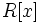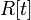# Polynomial ring

## Definition for commutative rings

### Definition with symbols

Let$R$ denote a commutative unital ring. The, the polynomial ring over$R$ in one variable, denoted as$R[x]$ where$x$ is termed the indeterminate, is defined as the ring of formal polynomials in$x$ with coefficients in$R$.

Note that we could choose any letter for the indeterminate in place of$x$ and our notation would change accordingly. For instance, if we use the letter$t$ for the indeterminate, the ring is denoted$R[t]$.

## Extra structure

The polynomial ring over any commutative unital ring$R$ is, first and foremost, a commutative unital ring. However, it has a number of additional structures, some of which are described below.

### As an algebra over the original ring

The polynomial ring$R[x]$ is a$R$-algebra. In fact, any$R$-algebra generated by one element over$R$, is a quotient, as a$R$-algebra, of$R[x]$.

The polynomial ring comes with a natural gradation. The$d^{th}$ graded component of the polynomial ring is the$R$-span of$x^d$.

In fact, this makes$R[x]$ a connected graded$R$-algebra.

### As a filtered ring

The polynomial ring comes with a natural filtration. The$d^{th}$ filtered component of the polynomial ring is the subgroup comprising the polynomials of degree at most$d$. This is the filtration corresponding to the gradation described above, and makes$R[x]$ a connected filtered$R$-algebra.

## Functoriality

The polynomial ring can be viewed as a functor in any of the following senses:

## Preservation of structure and properties

For many properties of commutative unital rings, it is true that when we pass to the polynomial ring, the property is preserved. However, this is not true for all properties of interest. For a list of properties that are preserved on passing to the polynomial ring, refer:

## Related notions

### Variations that are the same over a field of characteristic zero

Some important notions include: